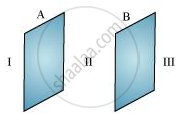# Two large, thin metal plates are parallel and close to each other. On their inner faces, the plates have surface charge densities of opposite signs and of magnitude 17.0 × 10−22 C/m2. What is E: - Physics

Numerical

Two large, thin metal plates are parallel and close to each other. On their inner faces, the plates have surface charge densities of opposite signs and of magnitude 17.0 × 10−22 C/m2. What is E: (a) in the outer region of the first plate, (b) in the outer region of the second plate, and (c) between the plates?

#### Solution

The situation is represented in the following figure.A and B are two parallel plates close to each other. Outer region of plate A is labelled as I, the outer region of plate B is labelled as III, and the region between the plates, A and B, is labelled as II.

Charge density of plate A, σ = 17.0 × 10−22 C/m2

Charge density of plate B, σ = −17.0 × 10−22 C/m2

In regions, I and III, electric field E is zero. This is because the charge is not enclosed by the respective plates.

Electric field E in region II is given by the relation,

"E" = sigma/in_0

Where,

0 = Permittivity of free space = 8.854 × 10−12 N−1 Cm−2

∴ "E" = (17.0 xx 10^-22)/(8.854 xx 10^-12)

= 1.92 × 10−10 N/C

Therefore, the electric field between the plates is 1.92 × 10−10 N/C.

Concept: Continuous Distribution of Charges
Is there an error in this question or solution?

#### APPEARS IN

NCERT Physics Part 1 and 2 Class 12
Chapter 1 Electric Charges and Fields
Exercise | Q 1.24 | Page 48
NCERT Class 12 Physics Textbook
Chapter 1 Electric Charge and Fields
Exercise | Q 24 | Page 48

Share